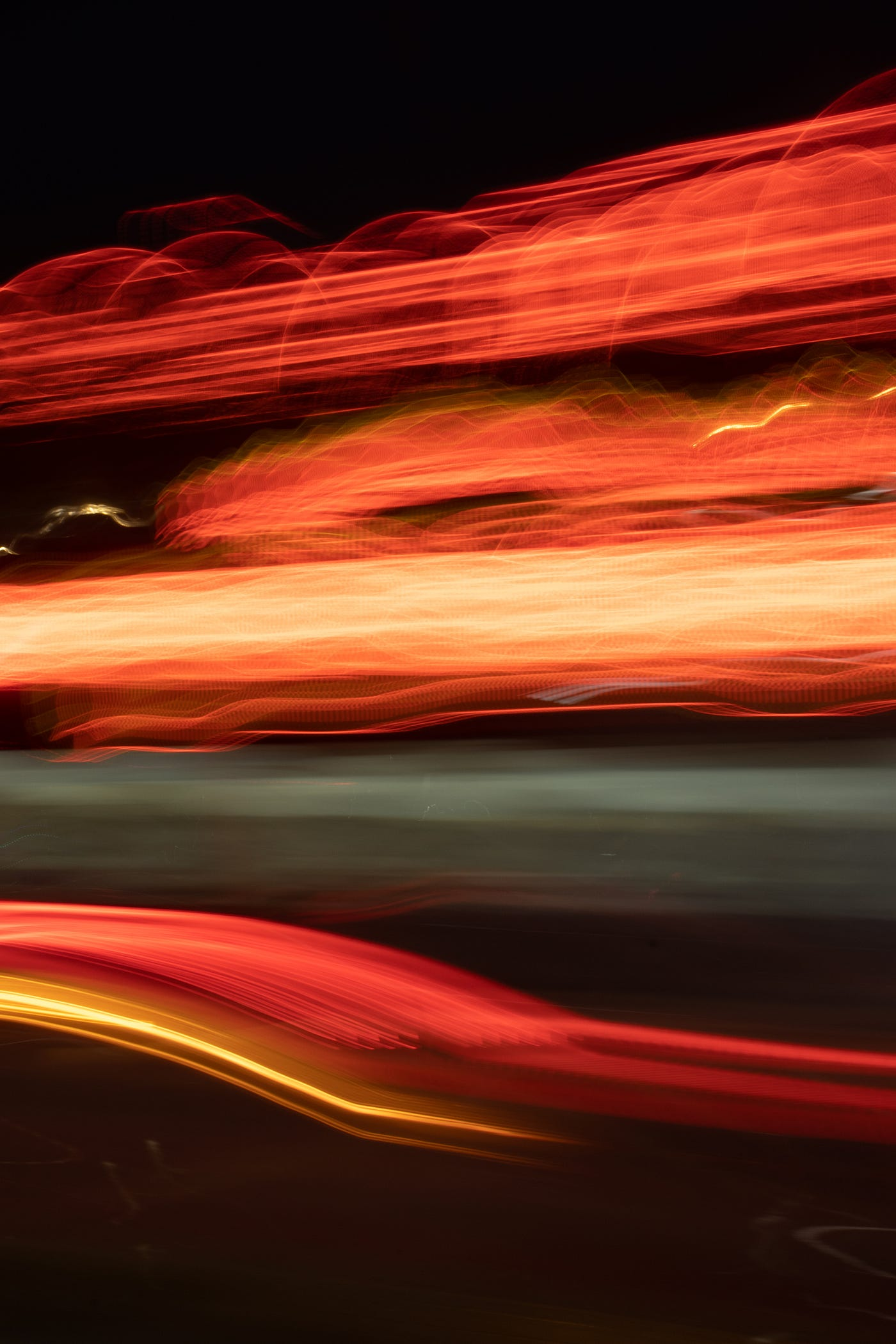# Faster R-CNN Object Detection with Region Proposal NetworksPhoto by Melyna Valle on Unsplash

# Architecture

The Faster R-CNN architecture consists of the RPN as a region proposal algorithm and the Fast R-CNN as a detector network.

• First of all, the model gets the input image and through the backbone CNN gets the feature map. Besides test time efficiency, another key reason using an RPN as a proposal generator makes sense is the advantages of weight sharing between the RPN backbone and the Fast R-CNN detector backbone.
• Next, the bounding box proposals from the RPN are used to pool features from the backbone feature map. This is done by the ROI pooling layer. The ROI pooling layer, in essence, works by a) Taking the region corresponding to a proposal from the backbone feature map; b) Dividing this region into a fixed number of sub-windows; c) Performing max-pooling over these sub-windows to give a fixed size output.
• The output from the ROI pooling layer has a size of (N, 7, 7, 512) where N is the number of proposals from the region proposal algorithm. After passing them through two fully connected layers, the features are fed into the sibling classification and regression branches.

## RPN(Region Proposal Networks)

An RPN is a fully convolutional network that simultaneously predicts object bounds and objectness scores at each position. The RPN is trained end-to-end to generate high-quality region proposals, which are used by Fast R-CNN for detection.
A Region Proposal Network (RPN) takes an image (of any size) as input and outputs a set of rectangular object proposals, each with an objectness score.

RPN has a classifier and a regressor. To generate region proposals, slide a small network over the convolutional feature map output by the last shared convolutional layer. This small network takes as input an n*n spatial window of the input convolutional feature map. Each sliding window is mapped to a lower-dimensional feature. This feature is fed into two sibling fully-connected layers — a box-regression layer (reg) and a box-classification layer (cls).
Classifier determines the probability of a proposal having the target object. Regression regresses the coordinates of the proposals.

## Anchors

At each sliding-window location, simultaneously predict multiple region proposals, where the number of maximum possible proposals for each location is denoted as k.
Anchor is the central point of the sliding window. For the ZF Model which was an extension of AlexNet, the dimensions are 256-d and for VGG-16, it was 512-d.
For those who don’t know, aspect ratio = width of image/height of the image, scale is the size of the image. The developers chose 3 scales and 3 aspect-ratio. So, a total of 9 proposals are possible for each pixel, this is how the value of k is decided, K=9 for this case, k being the number of anchors. For the whole image, a number of anchors are W*H*K.

These anchors are assigned labels based on two factors:

1. The anchors with the highest Intersection-over-union overlap with a ground truth box.
2. The anchors with Intersection-Over-Union Overlap are higher than 0.7.

## Multi-Scale

• The images are resized at multiple scales, and feature maps are computed for each scale. This way is often useful but is time-consuming.
• Use sliding windows of multiple scales (and/or aspect ratios) on the feature maps
Note
: Because of this multi-scale design based on anchors, we can simply use the convolutional features computed on a single-scale image, as is also done by the Fast R-CNN detector. The design of multiscale anchors is a key component for sharing features without extra cost for addressing scales.

## RPN Loss

For training RPNs, we assign a binary class label (of being an object or not) to each anchor.

i → Index of anchor, p → probability of being an object or not, t →vector of 4 parameterized coordinates of the predicted bounding box, * represents ground truth box. L for cls represents Log Loss over two classes.
p* with regression term in the loss function ensures that if and only if the object is identified as yes, then only regression will count, otherwise p* will be zero, so the regression term will become zero in the loss function.

Ncls and Nreg are the normalizations. Default λ is 10 by default and is done to scale the classifier and regressor on the same level.

• Detection with a VGG RPN takes 198ms compared to the 1.8 seconds of Selective Search.
• In ablation studies to observe the importance of scale and aspect ratios of anchor boxes, the authors find that using 3 scales with a single aspect ratio works almost as well as 3 scales and 3 aspect ratios. Depending on the task and the dataset, these ratios and scales can be modified. Using a single anchor at each location causes the mAP to drop considerably.

references:

 Shaoqing Ren, Kaiming He, Ross Girshick, and Jian Sun, Faster R-CNN: Towards Real-Time Object Detection with Region Proposal Networks, NIPS’15 Proceedings
 Girshick, Ross et al. “Rich Feature Hierarchies for Accurate Object Detection and Semantic Segmentation.” 2014 IEEE Conference on Computer Vision and Pattern Recognition (2014)
 Girshick, Ross. “Fast R-CNN.” 2015 IEEE International Conference on Computer Vision (ICCV) (2015)
 http://www.telesens.co/2018/03/11/object-detection-and-classification-using-r-cnns/

--

--